# Eckart conditions

In chemistry and physics, the Eckart conditions, named after Carl Eckart, sometimes referred to as Sayvetz conditions, simplify the nuclear motion (rovibrational) Schrödinger equation of a molecule. The rovibrational Schrödinger equation arises in the second step of the Born-Oppenheimer (BO) approximation.

The first step of the BO approximation yields a nuclear potential energy. This potential is generated by solution of the electronic Schrödinger equation of the molecule for different nuclear constellations. The potential energy, which is a function of the nuclear coordinates, enters the time-independent Schrödinger equation for the "motion" of the nuclei—the second step of the BO approximation. The eigenvalues obtained from the solution of the nuclear Schrödinger equation are the energies observed in the infrared and microwave spectra of the molecule. Microwave and infrared spectra are associated with rotations and vibrations of the nuclei in the molecule.

In undergraduate texts it is usually assumed—but not proved—that rotations and vibrations can be separated, i.e., that nuclear wave functions can be factorized as,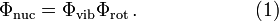$\Phi_\mathrm{nuc} = \Phi_\mathrm{vib}\Phi_\mathrm{rot} \,. \qquad\qquad\qquad(1)$

In these texts the coordinates are never made explicit. It is left to the reader to decide what the coordinates are that the wave functions depend on: space-fixed or body-fixed? If the latter, in what way are they fixed to the body, i.e., to the molecule? Eckart was the first to derive the coordinates that give the separation of the external (rotational and translational) motions from the internal (vibrational) motions. He found that the factorization is never exact, as there are always non-vanishing vibration-rotation coupling terms, the so-called Coriolis terms. Although the rotational and vibrational motions of the nuclei in a molecule cannot be fully separated, the Eckart conditions minimize the coupling between the two kinds of motion and accordingly make neglect of coupling a lesser approximation.

##  Rotational and translational Eckart conditions

The Eckart conditions can only be formulated for semi-rigid molecules, which are molecules with a potential energy surface V(R1, R2,..RN) that has a well-defined minimum for a set of nuclear positions RA0 ($A=1,\ldots, N$). Tunneling of the nuclei through potential barriers is excluded.

The equilibrium coordinates of the nuclei—with respective masses MA—are expressed with respect to an orthonormal principal axes frame with origin in the center of mass in the molecule. By definition, principal axes are eigenvectors of the inertia tensor, which is a symmetric 3×3 tensor. Accordingly, the equilibrium coordinates satisfy the relations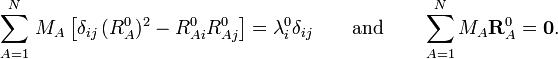$\sum_{A=1}^N\, M_A\,\big[\delta_{ij}\,(R_A^0)^2 - R^0_{Ai} R^0_{Aj}\big] = \lambda^0_i \delta_{ij} \qquad\mathrm{and}\qquad \sum_{A=1}^N M_A \mathbf{R}_A^0 = \mathbf{0}.$

Here λi0 is a principal inertia moment of the equilibrium molecule. The N triplets of real numbers RA0 = (RA10, RA20, RA30) satisfying these conditions, enter the theory as a known set of constant parameters.

Following Biedenharn and Louck we introduce an orthonormal body-fixed frame, the Eckart frame,$\vec{\mathbf{F}} \equiv \{ \vec{f}_1, \vec{f}_2, \vec{f}_3\} .$

If an observer were tied to the Eckart frame, which—following the molecule—rotates and translates in space, (s)he would constantly see the molecule in its equilibrium geometry, provided the nuclei are at the points,$\vec{R}_A^0 \equiv \vec{\mathbf{F}}\, \cdot\, \mathbf{R}_A^0 =\sum_{i=1}^3 \vec{f}_i\, R^0_{Ai},\quad A=1,\ldots,N .$.

The motion of the Eckart frame describes the external (translational and rotational) motion of the equilibrium molecule. Possible internal motion of the nuclei away from their equilibrium points is the molecular vibration. The Eckart frame is a non-inertial frame, hence one can expect pseudo (non-inertial) forces to act on the nuclei. Indeed, centrifugal and Coriolis forces will appear.

Let the elements of RA be the coordinates with respect to the Eckart frame of the position vector of nucleus A ($A=1,\ldots, N$). Since we take the origin of the Eckart frame in the instantaneous center of mass, the following relation holds,$\sum_A M_A \mathbf{R}_A = \mathbf{0}.$

We define displacement coordinates$\mathbf{d}_A\equiv\mathbf{R}_A-\mathbf{R}^0_A$.

Clearly the displacement coordinates satisfy the translational Eckart conditions,$\sum_{A=1}^N M_A \mathbf{d}_A = 0 .$

The rotational Eckart conditions for the displacements are: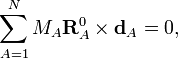$\sum_{A=1}^N M_A \mathbf{R}^0_A \times \mathbf{d}_A = 0,$

where × indicates a cross product. These rotational conditions follow from the specific construction of the Eckart frame vectors$\vec{f}_i$, see Biedenharn and Louck, loc. cit., page 538, for the details of their construction and how this construction leads to the rotational Eckart conditions.

Finally, notice that an Eckart frame becomes a principal axes frame in the case that the molecule is a rigid rotor, that is, when all N displacement vectors are zero, which occurs when the molecule is not vibrating.

## Separation of external and internal coordinates

The N position vectors$\vec{R}_A$ of the nuclei in a molecule constitute a 3N dimensional linear space R3N: the nuclear configuration space. From the Eckart conditions follows that the nuclear configuration space is an orthogonal direct sum. This direct sum decomposition of the nuclear configuration space gives a separation of the nuclear coordinates in vibration coordinates on the one hand and rotation/translation coordinates on the other.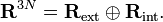$\mathbf{R}^{3N} = \mathbf{R}_\textrm{ext}\oplus\mathbf{R}_\textrm{int}.$

The elements of the 3N−6 dimensional subspace Rint are referred to as internal coordinates, because they are invariant under overall translation and rotation of the molecule and, thus, depend only on the internal (vibrational) motions. The elements of the 6-dimensional subspace Rext are referred to as external coordinates, because they are associated with the overall translation and rotation of the molecule.

To show the direct sum decomposition, we define first a basis for Rext and introduce the following 6 vectors:\begin{align} \vec{s}^A_{i} \equiv \vec{f}_i \quad\hbox{and}\quad \vec{s}^A_{i+3} \equiv \vec{f}_i \times\vec{R}_A^0,\qquad i=1,2,3,\quad A=1,\ldots,N\\ \end{align}

An orthogonal, unnormalized, basis for Rext is then,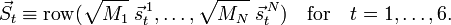$\vec{S}_t \equiv \operatorname{row}(\sqrt{M_1}\;\vec{s}^{\,1}_{t}, \ldots, \sqrt{M_N} \;\vec{s}^{\,N}_{t}) \quad\mathrm{for}\quad t=1,\ldots, 6.$

A mass-weighted displacement vector is$\vec{D} \equiv \operatorname{col}(\sqrt{M_1}\;\vec{d}^{\,1}, \ldots, \sqrt{M_N}\;\vec{d}^{\,N}) \quad\mathrm{with}\quad \vec{d}^{\,A} \equiv \vec{\mathbf{F}}\cdot \mathbf{d}_A .$

For i=1,2,3,$\vec{S}_i \cdot \vec{D} = \sum_{A=1}^N \; M_A \vec{s}^{\,A}_i \cdot \vec{d}^{\,A} =\sum_{A=1}^N M_A d_{Ai} = 0,$

where the zero follows because of the translational Eckart conditions. For i=4,5,6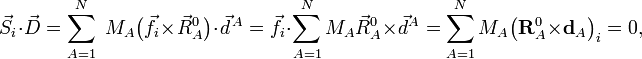$\, \vec{S}_i \cdot \vec{D} = \sum_{A=1}^N \; M_A \big(\vec{f}_i \times\vec{R}_A^0\big) \cdot \vec{d}^{\,A}=\vec{f}_i \cdot \sum_{A=1}^N M_A \vec{R}_A^0 \times\vec{d}^A = \sum_{A=1}^N M_A \big( \mathbf{R}_A^0 \times \mathbf{d}_A\big)_i = 0,$

where the zero follows because of the rotational Eckart conditions. We conclude that the displacement vector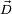$\vec{D}$ belongs to the orthogonal complement of Rext, and hence that a mass-weighted displacement vector is an internal vector.

A basis for the internal space is obtained by introduction of 3N−6 linearly independent vectors$\vec{Q}_r \equiv \operatorname{row}(\frac{1}{\sqrt{M_1}}\;\vec{q}_r^{\,1}, \ldots, \frac{1}{\sqrt{M_N}}\;\vec{q}_r^{\,N}), \quad\mathrm{for}\quad r=1,\ldots, 3N-6.$

The vectors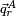$\vec{q}^A_r$ could be Wilson's s-vectors or could be obtained in the harmonic approximation by diagonalizing the Hessian of V. We next introduce internal (vibrational) modes,$q_r \equiv \vec{Q}_r \cdot \vec{D} = \sum_{A=1}^N \vec{q}^A_r \cdot \vec{d}^{\,A} \quad\mathrm{for}\quad r=1,\ldots, 3N-6.$

The physical meaning of qr depends on the vectors$\vec{q}^A_r$. For instance, qr could be a symmetric stretching mode, in which two C—H bonds are simultaneously stretched and contracted.

We already saw that the corresponding external modes are zero because of the Eckart conditions,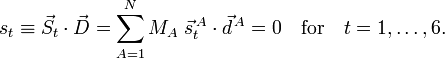$s_t \equiv \vec{S}_t \cdot \vec{D} = \sum_{A=1}^N M_A \;\vec{s}^{\,A}_t \cdot \vec{d}^{\,A} = 0 \quad\mathrm{for}\quad t=1,\ldots, 6.$

## Overall translation and rotation

As we stated above, the internal (vibrational) coordinates are invariant under translation and infinitesimal rotation of the equilibrium molecule, hence their name. We will now show that these invariances hold if and only if the Eckart conditions apply.

An overall translation of the reference ( = equilibrium) molecule is given by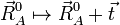$\vec{R}_{A}^0 \mapsto \vec{R}_{A}^0 + \vec{t}$'

for any arbitrary 3-vector$\vec{t}$. An infinitesimal rotation of the molecule is given by$\vec{R}_A^0 \mapsto \vec{R}_A^0 + \Delta\phi \; ( \vec{n}\times \vec{R}_A^0)$

where Δφ is an infinitesimal angle, Δφ >> (Δφ)², and$\vec{n}$ is an arbitrary unit vector. From the orthogonality of$\vec{Q}_r$ to the external space follows that the$\vec{q}^A_r$ satisfy$\sum_{A=1}^N \vec{q}^{\,A}_r = \vec{0} \quad\mathrm{and}\quad \sum_{A=1}^N \vec{R}^0_A\times \vec{q}^A_r = \vec{0}.$

Now, under translation$q_r \mapsto \sum_A\vec{q}^{\,A}_r \cdot(\vec{d}^A - \vec{t}) = q_r - \vec{t}\cdot\sum_A \vec{q}^{\,A}_r = q_r.$

Clearly,$\vec{q}^A_r$ is invariant under translation if and only if$\sum_A \vec{q}^{\,A}_r = \vec{0},$

because the vector$\vec{t}$ is arbitrary. So, the translational Eckart conditions imply the translational invariance of the vectors belonging to internal space and conversely. Under rotation we have,$q_r \mapsto \sum_A\vec{q}^{\,A}_r \cdot \big(\vec{d}^A - \Delta\phi \; ( \vec{n}\times \vec{R}_A^0) \big) = q_r - \Delta\phi \; \vec{n}\cdot\sum_A \vec{R}^0_A\times\vec{q}^{\,A}_r = q_r.$

Rotational invariance follows if and only if$\sum_A \vec{R}^0_A\times\vec{q}^{\,A}_r =\vec{0}.$

The external modes, on the other hand, are not invariant and it is not difficult to show that they change under translation as follows:\begin{align} s_i &\mapsto s_i + M \vec{f}_i \cdot \vec{t} \quad \mathrm{for}\quad i=1,2,3 \\ s_i &\mapsto s_i \quad \mathrm{for}\quad i=4,5,6, \\ \end{align}

where M is the total mass of the molecule. They change under infinitesimal rotation as follows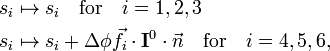\begin{align} s_i &\mapsto s_i \quad \mathrm{for}\quad i=1,2,3 \\ s_i &\mapsto s_i + \Delta \phi \vec{f}_i \cdot \mathbf{I}^0\cdot \vec{n} \quad \mathrm{for}\quad i=4,5,6, \\ \end{align}

where I0 is the inertia tensor of the equilibrium molecule. This behavior shows that the first three external modes describe the overall translation of the molecule, while the modes 4, 5, and, 6 describe the overall rotation.

## Vibrational energy

The vibrational energy of the molecule can be written in terms of coordinates with respect to the Eckart frame as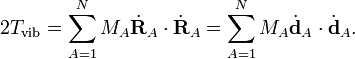$2T_\mathrm{vib} = \sum_{A=1}^N M_A \dot{\mathbf{R}}_A\cdot \dot{\mathbf{R}}_A = \sum_{A=1}^N M_A \dot{\mathbf{d}}_A\cdot \dot{\mathbf{d}}_A.$

Because the Eckart frame is non-inertial (moves non-uniformly in space), the total kinetic energy comprises also centrifugal and Coriolis energies. These kinetic energy terms couple the vibrational with the rotational motions. See Watson's Hamiltonian for the explicit form of the coupling terms.

In this section we will ignore the coupling. Quantum mechanically the neglect of coupling implies that the rotation/translation Schrödinger equation (depending on the external coordinates) can be solved separately from the vibrational Schrödinger equation, which depends solely on the internal coordinates. The nuclear wave function then gets the product form of equation (1). This is an important computational and conceptual simplification, that however, amounts to neglect of some observable effects on the vibration spectrum.

The vibrational energy is written in terms of the displacement coordinates, which are linearly dependent because they are "contaminated" by the 6 external coordinates, which are zero, i.e., the dA's satisfy 6 linear relations. An essential result is the fact that it is possible to write the vibrational energy solely in terms of the internal coordinates qr (r =1, ..., 3N−6). In order to exhibit this result, we write the different coordinates in terms of the displacements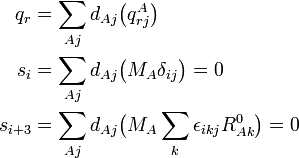\begin{align} q_r = \sum_{Aj} d_{Aj}& \big( q^A_{rj} \big) \\ s_i = \sum_{Aj} d_{Aj}& \big( M_A \delta_{ij} \big) =0 \\ s_{i+3} = \sum_{Aj} d_{Aj}& \big( M_A \sum_k \epsilon_{ikj} R^0_{Ak} \big)=0 \\ \end{align}

The parenthesized expressions define a matrix B relating the internal and external modes to the displacements. The matrix B may be partitioned in an internal (3N−6 x 3N) and an external (6 x 3N) part,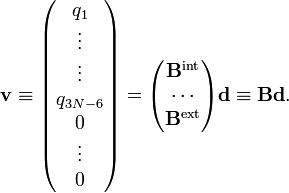$\mathbf{v}\equiv \begin{pmatrix} q_1 \\ \vdots \\ \vdots \\ q_{3N-6} \\ 0 \\ \vdots \\ 0\\ \end{pmatrix} = \begin{pmatrix} \mathbf{B}^\mathrm{int} \\ \cdots \\ \mathbf{B}^\mathrm{ext} \\ \end{pmatrix} \mathbf{d} \equiv \mathbf{B} \mathbf{d}.$

We define the matrix M by$\mathbf{M} \equiv \operatorname{diag}(\mathbf{M}_1, \mathbf{M}_2, \ldots,\mathbf{M}_N) \quad\textrm{and}\quad \mathbf{M}_A\equiv \operatorname{diag}(M_A, M_A, M_A)$

and from the relations given in the previous sections follow the matrix relations$\mathbf{B}^\mathrm{ext} \mathbf{M}^{-1} (\mathbf{B}^\mathrm{ext})^\mathrm{T} = \operatorname{diag}(N_1,\ldots, N_6) \equiv\mathbf{N},$

and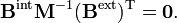$\mathbf{B}^\mathrm{int} \mathbf{M}^{-1} (\mathbf{B}^\mathrm{ext})^\mathrm{T} = \mathbf{0}.$

We define$\mathbf{G} \equiv \mathbf{B}^\mathrm{int} \mathbf{M}^{-1} (\mathbf{B}^\mathrm{int})^\mathrm{T}.$

By using the rules for block matrix multiplication we can show that$(\mathbf{B}^\mathrm{T})^{-1} \mathbf{M} \mathbf{B}^{-1} = \begin{pmatrix} \mathbf{G}^{-1} && \mathbf{0} \\ \mathbf{0} && \mathbf{N}^{-1} \end{pmatrix},$

where G−1 is of dimension (3N−6 x 3N−6) and N−1 is (6 x 6). The kinetic energy becomes$2T_\mathrm{vib} = \dot{\mathbf{d}}^\mathrm{T} \mathbf{M} \dot{\mathbf{d}} = \dot{\mathbf{v}}^\mathrm{T}\; (\mathbf{B}^\mathrm{T})^{-1} \mathbf{M} \mathbf{B}^{-1}\; \dot{\mathbf{v}} = \sum_{r, r'=1}^{3N-6} (G^{-1})_{r r'} \dot{q}_r \dot{q}_{r'}$

where we used that the last 6 components of v are zero. This form of the kinetic energy of vibration enters Wilson's GF method. It is of some interest to point out that the potential energy in the harmonic approximation can be written as follows$2V_\mathrm{harm} = \mathbf{d}^\mathrm{T} \mathbf{H} \mathbf{d} = \mathbf{v}^\mathrm{T} (\mathbf{B}^\mathrm{T})^{-1} \mathbf{H} \mathbf{B}^{-1} \mathbf{v} = \sum_{r, r'=1}^{3N-6} F_{r r'} q_r q_{r'},$

where H is the Hessian of the potential in the minimum and F, defined by this equation, is the F matrix of the GF method.

##  Relation to the harmonic approximation

In the harmonic approximation to the nuclear vibrational problem, expressed in displacement coordinates, one must solve the generalized eigenvalue problem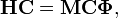$\mathbf{H}\mathbf{C} = \mathbf{M} \mathbf{C} \boldsymbol{\Phi},$

where H is a 3N x 3N symmetric matrix of second derivatives of the potential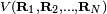$V(\mathbf{R}_1, \mathbf{R}_2,\ldots, \mathbf{R}_N)$. H is the Hessian matrix of V in the equilibrium$\mathbf{R}_1^0,\ldots, \mathbf{R}_N^0$. The diagonal matrix M contains the masses on the diagonal. The diagonal matrix$\boldsymbol{\Phi}$ contains the eigenvalues, while the columns of C contain the eigenvectors.

It can be shown that the invariance of V under simultaneous translation over t of all nuclei implies that vectors T = (t, ... , t) are in the kernel of H. From the invariance of V under an infinitesimal rotation of all nuclei around s it can be shown that also the vectors S = (s x R10, ..., s x RN0) are in the kernel of H :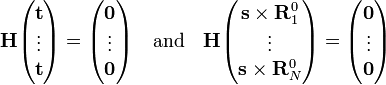$\mathbf{H} \begin{pmatrix} \mathbf{t} \\ \vdots\\ \mathbf{t} \end{pmatrix} = \begin{pmatrix} \mathbf{0} \\ \vdots\\ \mathbf{0} \end{pmatrix} \quad\mathrm{and}\quad \mathbf{H} \begin{pmatrix} \mathbf{s}\times \mathbf{R}_1^0 \\ \vdots\\ \mathbf{s}\times \mathbf{R}_N^0 \end{pmatrix} = \begin{pmatrix} \mathbf{0} \\ \vdots\\ \mathbf{0} \end{pmatrix}$

Thus, 6 columns of C corresponding to eigenvalue zero, are determined algebraically. (If the generalized eigenvalue problem is solved numerically, one will find in general 6 linearly independent linear combinations of S and T). The eigenspace corresponding to eigenvalue zero is at least of dimension 6 (often it is exactly of dimension 6, since the other eigenvalues, which are force constants, are never zero for molecules in their ground state). Thus, T and S correspond to the overall (external) motions: translation and rotation, respectively. They are zero-energy modes because space is homogeneous (force-free) and isotropic (torque-free).

By the definition in this article the non-zero frequency modes are internal modes, since they are within the orthogonal complement of Rext. The generalized orthogonalities: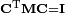$\mathbf{C}^\mathrm{T} \mathbf{M} \mathbf{C} = \mathbf{I}$ applied to the "internal" (non-zero eigenvalue) and "external" (zero-eigenvalue) columns of C are equivalent to the Eckart conditions.